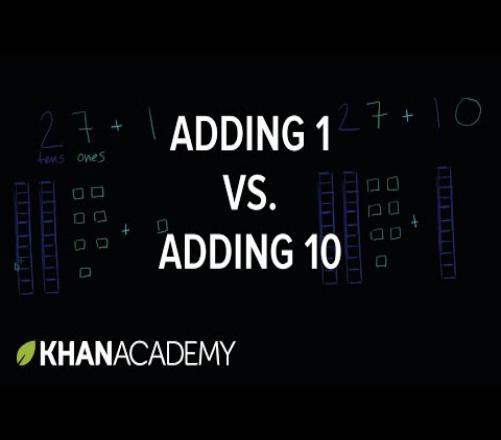Approved & Edited by ProProfs Editorial Team
At ProProfs Quizzes, our dedicated in-house team of experts takes pride in their work. With a sharp eye for detail, they meticulously review each quiz. This ensures that every quiz, taken by over 100 million users, meets our standards of accuracy, clarity, and engagement.
| Written by Jaksiboy
J
Jaksiboy
Community Contributor
Total Contribution - 924 | Total attempts - 12,123,294
Questions: 10 | Attempts: 123SettingsPrinciples apply in mathematics and this principle hasn’t changed to date when given a set of an equation to solve, taking and applying this principle gets the job done easily. Take a quiz using one of this principle of mathematics called addition and test your knowledge.

• 1.

### Taking the number four add it to twelve plus two, you will arrive at a certain result within the range of twenty, what is your final answer? Which one do you like?

• A.

Thirteen

• B.

Fourteen

• C.

Sixteen

• D.

Eighteen

D. Eighteen
Explanation
By adding four to twelve and then adding two, we get a total of eighteen.

Rate this question:

• 2.

### Add seven, plus three plus zero plus two plus zero and state your final answers which should be in the range of one to twenty.

• A.

Thirteen

• B.

Nine

• C.

Eleven

• D.

Twelve

D. Twelve
Explanation
The given question asks to add seven, three, zero, two, and zero. Adding these numbers together gives a total of twelve.

Rate this question:

• 3.

### Take twelve and add it to four plus two plus zero plus one, what is your final answer after adding these figures?

• A.

Twenty

• B.

Eighteen

• C.

Fourteen

• D.

Nineteen

D. Nineteen
Explanation
The question asks to add twelve to four plus two plus zero plus one. When we add these figures, we get a sum of nineteen.

Rate this question:

• 4.

### Take number seven add to four plus three and one plus zero plus one and give your final answer within the range of twenty?

• A.

Fourteen

• B.

Thirteen

• C.

Sixteen

• D.

Fifteen

C. Sixteen
Explanation
To find the final answer within the range of twenty, we need to add the numbers given in the question: seven, four, three, one, zero, and one. Adding these numbers together gives us a total of sixteen. Since sixteen is within the range of twenty, it is the correct answer.

Rate this question:

• 5.

### Add three plus four plus seven and give your final answer which should be within the range of one to twenty.

• A.

Ten

• B.

Fourteen

• C.

Fifteen

• D.

Four

B. Fourteen
Explanation
The correct answer is Fourteen because when we add three, four, and seven together, we get a sum of fourteen, which falls within the given range of one to twenty.

Rate this question:

• 6.

### Take a particular number and add it to another to arrive at sixteen, now what number do you need to add together to get sixteen?

• A.

Ten plus six

• B.

Nine plus six

• C.

Seven plus eight

• D.

Eight plus six

A. Ten plus six
Explanation
To arrive at sixteen, the question asks for a number that needs to be added to another number. The only option that satisfies this condition is "Ten plus six" as adding ten to six equals sixteen.

Rate this question:

• 7.

### Add few number together to get accurate of figure twenty, what number do you need to add together for this?

• A.

Three plus sixteen

• B.

Five plus four plus two

• C.

Ten plus five

• D.

Zero plus ten plus ten

D. Zero plus ten plus ten
Explanation
To get an accurate figure of twenty, you need to add zero plus ten plus ten. This is because the sum of these numbers is 20.

Rate this question:

• 8.

### Add numbers together to get the total of zero within the range of zero to twenty, what figures do you need to add?

• A.

Zero plus zero

• B.

Two plus twelve

• C.

One plus sixteen

• D.

Zero plus two

A. Zero plus zero
Explanation
To get a total of zero, you need to add numbers that cancel each other out. In this case, zero plus zero equals zero. The other options, two plus twelve, one plus sixteen, and zero plus two, all result in numbers that are greater than zero. Therefore, the correct answer is zero plus zero.

Rate this question:

• 9.

### Three plus five plus seven will give a particular number if we add two to it, what is the final result?

• A.

Nineteen

• B.

Ten

• C.

Twelve

• D.

Seventeen

D. Seventeen
Explanation
If we add two to the sum of three, five, and seven, we get a final result of seventeen.

Rate this question:

• 10.

### Zero plus zero plus five plus one will give a whole number, add the three to it and what will it give us finally?

• A.

Fourteen

• B.

Twenty

• C.

Ten

• D.

NineBack to top